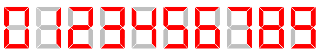# HW2F - Editorial

Practice
Source

Author: SQU_Test

DIFFICULTY:
Easy

PREREQUISITES:
Basic observations.

PROBLEM:
You have a large electronic board which can display up to 998244353 decimal digits. The digits are displayed in the same way as on different electronic alarm clocks: each place for a digit consists of 7 segments which can be turned on and off to compose different digits. The following picture describes how you can display all 10 decimal digits:As you can see, different digits may require different number of segments to be turned on. For example, if you want to display 1, you have to turn on 2 segments of the board, and if you want to display 8, all 7 segments of some place to display a digit should be turned on.

You want to display a really large integer on the board. Unfortunately, the board is bugged: no more than n segments can be turned on simultaneously. So now you wonder what is the greatest integer that can be displayed by turning on no more than n segments.

Your program should be able to process t different test cases.

EXPLANATION:
First of all, we don’t need to use any digits other than 1 and 7. If we use any other digit, it consists of 4 or more segments, so it can be replaced by two 1’s and the number will become greater. For the same reason we don’t need to use more than one 7: if we have two, we can replace them with three 1’s.
Obviously, it is always optimal to place 7 before 1. So, our number is either a sequence of 1’s, or a 7 and a sequence of 1’s. We should use 7 only if n is odd, because if n is even, it will decrease the number of digits in the result.

TIME COMPLEXITY:
Time complexity of the solution is O(n).

SOLUTIONS:

Setter’s Solution
``````T = int(input())
for t in range(T):
n = int(input())
if(n % 2 == 1):
print(7, end='')
n -= 3
while(n > 0):
print(1, end='')
n -= 2
print()
``````